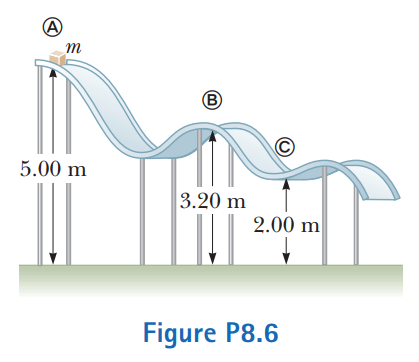# Problem: A block of mass m = 5.00 kg is released from point A and slides on the frictionless track shown in Figure P8.6. Determine(a) the block’s speed at points B and C and(b) the net work done by the gravitational force on the block as it moves from point A to point C.

⚠️Our tutors found the solution shown to be helpful for the problem you're searching for. We don't have the exact solution yet.

###### Problem Details

A block of mass m = 5.00 kg is released from point A and slides on the frictionless track shown in Figure P8.6. Determine(a) the block’s speed at points B and C and

(b) the net work done by the gravitational force on the block as it moves from point A to point C.